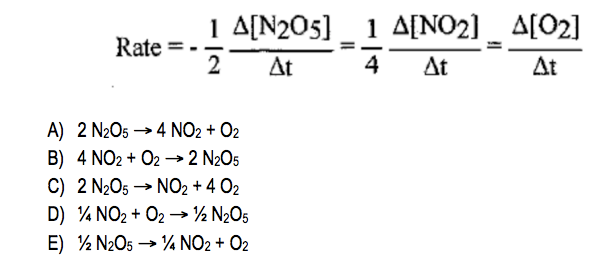Chemistry Practice Problems Average Rate of Reaction Practice Problems Solution: What is the balanced reaction for the following ra...

🤓 Based on our data, we think this question is relevant for Professor Mitchell's class at Truckee Meadows Community College.

# Solution: What is the balanced reaction for the following rate relationships?

###### Problem

What is the balanced reaction for the following rate relationships?Average Rate of Reaction

Average Rate of Reaction

#### Q. What is the average rate of formation of I2? Express your answer to three decimal places and include the appropriate units.Now consider the following ...

Solved • Tue Mar 17 2020 18:13:08 GMT-0400 (EDT)

Average Rate of Reaction

#### Q. What is the rate for the formation of Cu(s) in the reaction Cu2+(aq) + H2(g) ⇌ Cu(s) + 2 H+(aq) if Δ[H+] / Δt = 1.2 x 10 -3 ?1. 6 x 10-42. 2.4 x 10-33...

Solved • Fri Apr 07 2017 13:21:58 GMT-0400 (EDT)

Average Rate of Reaction

#### Q. Consider the following reaction:6NaOH + Al2O3 + 12HF → 2Na3AlF6 + 9H2OAt a given time, water is being produced at a rate of 0.54 M/min for this reacti...

Solved • Mon Aug 15 2016 12:54:04 GMT-0400 (EDT)

Average Rate of Reaction

#### Q. Given the following balanced equation, if the rate of Cl2 loss is 4.24 × 10-2 M/s, what is the rate of formation of NOCl?

Solved • Tue Feb 02 2016 15:49:07 GMT-0500 (EST)